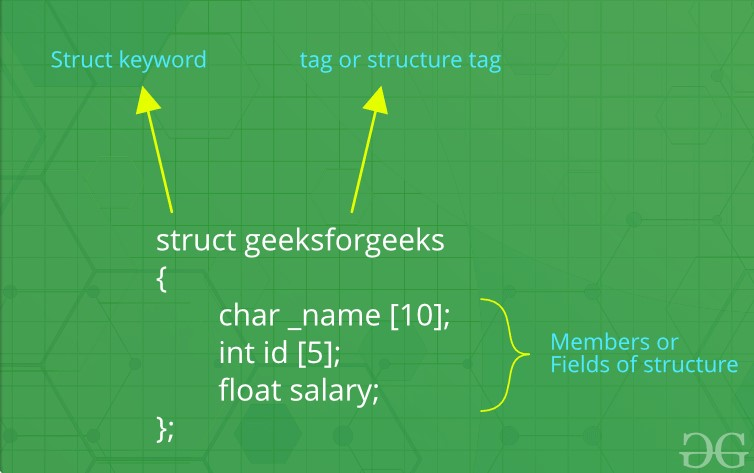# Structures in C++

We often come around situations where we need to store a group of data whether of similar data types or non-similar data types. We have seen Arrays in C++ which are used to store set of data of similar data types at contiguous memory locations.

Unlike Arrays, Structures in C++ are user defined data types which are used to store group of items of non-similar data types.

What is a structure?

A structure is a user-defined data type in C/C++. A structure creates a data type that can be used to group items of possibly different types into a single type.Structures in C++

How to create a structure?

The ‘struct’ keyword is used to create a structure. The general syntax to create a structure is as shown below:

```struct structureName{
member1;
member2;
member3;
.
.
.
memberN;
};
```

Structures in C++ can contain two types of members:

• Data Member: These members are normal C++ variables. We can create a structure with variables of different data types in C++.
• Member Functions: These members are normal C++ functions. Along with variables, we can also include functions inside a structure declaration.

Example:

 `// Data Members ` `int` `roll; ` `int` `age; ` `int` `marks; ` `     `  `// Member Functions ` `void` `printDetails() ` `{ ` `    ``cout<<``"Roll = "``<

In the above structure, the data members are three integer variables to store roll number, age and marks of any student and the member function is printDetails() which is printing all of the above details of any student.

How to declare structure variables?

A structure variable can either be declared with structure declaration or as a separate declaration like basic types.

 `// A variable declaration with structure declaration. ` `struct` `Point ` `{ ` `   ``int` `x, y; ` `} p1;  ``// The variable p1 is declared with 'Point' ` ` `  ` `  `// A variable declaration like basic data types ` `struct` `Point ` `{ ` `   ``int` `x, y; ` `};  ` ` `  `int` `main() ` `{ ` `   ``struct` `Point p1;  ``// The variable p1 is declared like a normal variable ` `}`

Note: In C++, the struct keyword is optional before in declaration of a variable. In C, it is mandatory.

How to initialize structure members?
Structure members cannot be initialized with declaration. For example the following C program fails in compilation.
But is considered correct in C++11 and above.

 `struct` `Point ` `{ ` `   ``int` `x = 0;  ``// COMPILER ERROR:  cannot initialize members here ` `   ``int` `y = 0;  ``// COMPILER ERROR:  cannot initialize members here ` `};  `

The reason for above error is simple, when a datatype is declared, no memory is allocated for it. Memory is allocated only when variables are created.

Structure members can be initialized using curly braces ‘{}’. For example, following is a valid initialization.

 `struct` `Point ` `{ ` `   ``int` `x, y; ` `};  ` ` `  `int` `main() ` `{ ` `   ``// A valid initialization. member x gets value 0 and y ` `   ``// gets value 1.  The order of declaration is followed. ` `   ``struct` `Point p1 = {0, 1};  ` `}`

How to access structure elements?
Structure members are accessed using dot (.) operator.

 `#include ` `using` `namespace` `std; ` ` `  `struct` `Point { ` `    ``int` `x, y; ` `}; ` ` `  `int` `main() ` `{ ` `    ``struct` `Point p1 = { 0, 1 }; ` ` `  `    ``// Accessing members of point p1 ` `    ``p1.x = 20; ` `    ``cout << ``"x = "` `<< p1.x << ``", y = "` `<< p1.y; ` ` `  `    ``return` `0; ` `}`

Output:

```x = 20, y = 1
```

What is an array of structures?

Like other primitive data types, we can create an array of structures.

 `#include ` `using` `namespace` `std; ` ` `  `struct` `Point { ` `    ``int` `x, y; ` `}; ` ` `  `int` `main() ` `{ ` `    ``// Create an array of structures ` `    ``struct` `Point arr; ` ` `  `    ``// Access array members ` `    ``arr.x = 10; ` `    ``arr.y = 20; ` ` `  `    ``cout << arr.x << ``" "` `<< arr.y; ` `    ``return` `0; ` `} `

Output:

```10 20
```

What is a structure pointer?
Like primitive types, we can have pointer to a structure. If we have a pointer to structure, members are accessed using arrow ( -> ) operator instead of the dot (.) operator.

 `#include ` `using` `namespace` `std; ` ` `  `struct` `Point { ` `    ``int` `x, y; ` `}; ` ` `  `int` `main() ` `{ ` `    ``struct` `Point p1 = { 1, 2 }; ` ` `  `    ``// p2 is a pointer to structure p1 ` `    ``struct` `Point* p2 = &p1; ` ` `  `    ``// Accessing structure members using ` `    ``// structure pointer ` `    ``cout << p2->x << ``" "` `<< p2->y; ` `    ``return` `0; ` `}`

Output:

```1 2
```

What is structure member alignment?

In C++, a structure is the same as a class except for a few differences. The most important of them is security. A Structure is not secure and cannot hide its implementation details from the end user while a class is secure and can hide its programming and designing details. Learn more about the differences between Structures and Class in C++.

Rated as one of the most sought after skills in the industry, own the basics of coding with our C++ STL Course and master the very concepts by intense problem-solving.

My Personal Notes arrow_drop_upSenior Technical Content Engineer | GeeksforGeeks

If you like GeeksforGeeks and would like to contribute, you can also write an article using contribute.geeksforgeeks.org or mail your article to contribute@geeksforgeeks.org. See your article appearing on the GeeksforGeeks main page and help other Geeks.

Please Improve this article if you find anything incorrect by clicking on the "Improve Article" button below.

Improved By : nabhaninajm, shubham_singh

Article Tags :
Practice Tags :

31

Please write to us at contribute@geeksforgeeks.org to report any issue with the above content.